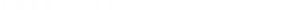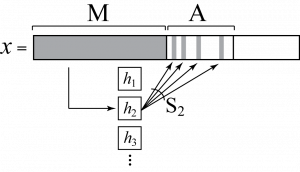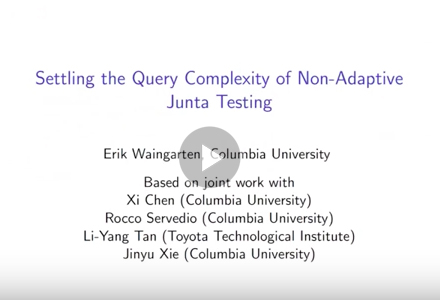# Paper by Columbia researchers named best paper at Computational Complexity ConferenceSettling the query complexity of non-adaptive junta testing” was named best paper at last week’s 32nd Computational Complexity Conference (CCC), the top venue for research in computational complexity theory, the branch of theoretical computer science that aims at understanding what makes certain problems computationally hard. The authors are five Columbia University researchers: Xi Chen, Rocco A. Servedio, Li-Yang Tan (now at Toyota Technological Institute in Chicago), Erik Waingarten, and Jinyu Xie. The last two authors are PhD students.

The paper gives the final answer to a well-studied question in property testing, an area of theoretical computer science: given an unknown Boolean function, how many nonadaptive queries are required to determine whether it is a k-junta versus far from every k-junta?

A k-junta is a function whose value is determined by a subset of at most k relevant variables, out of a potentially huge number of input variables. For example, a decision rule for determining whether a movie is a box office bomb or not (as a Boolean function) would ignore most input attributes—title, director, leading actor, genre, release date, running length, rating, etc.—to consider maybe only two: budget and box office receipts. A simple function such as this, depending on only two variables, is a 2-junta function; a function that looked at 100 attributes would be a 100-junta function.

A function that is a k-junta is relatively simple because it relies on only a small number (k) of variables; in such cases, dimension reduction techniques could potentially be used to remove extraneous variables. A function that is far from every k-junta, on the other hand, is determined by more than k variables and is in fact different from any k-junta on a large fraction of inputs; dimension reduction will not do much to simplify such a problem because the output depends on many variables.

Testing for the k-junta property, which is a helpful first step in exploratory data analysis, works by querying values of the function on inputs chosen by the testing algorithm. The smallest number of such queries required for nonadaptive algorithms—where queries are sent all in one simultaneous batch (as opposed to being sent one after the other, as occurs in adaptive algorithms where subsequent queries can depend on the answers obtained in response to earlier queries)—had been an open question until this paper, which now establishes that at least k1.5 nonadaptive queries are required. This result represents a dramatic improvement over previous lower bounds, and matches the upper bound of k1.5 given by Eric Blais in his 2008 paper Improved bounds for testing juntas.

The new lower bound was achieved by constructing a pair of hard-to-distinguish probability distributions supported on k-juntas and functions that are far from k-juntas, respectively. It is based on an idea of random indexing that the Columbia researchers have started exploring for a range of problems, applying it in particular to make progress in monotonicity testing (see Beyond Talagrand functions: new lower bounds for testing monotonicity and unateness). For k-juntas, the functions used in the paper are illustrated in the following figure:This figure shows how an input x in {0,1}n is evaluated by a function f used in the paper. Two disjoint subsets of randomly sampled variables, M and A, determine f(x) in the following way. Given x, one first interprets the variables in M as the index i of a function hi from a sequence of random functions, each of which depends only on a small subset of variables in A. Then f(x) is set to the value of hi evaluated on the relevant variables in the small subset of A (depicted by the four narrow shaded bands). By adjusting the size of A, f becomes either a k-junta or far from every k-junta, for some appropriate parameter k.

“Once we had the initial idea, the work went quickly; in less than a month, we had the result,” says Chen. “The surprise is that we could push it all the way to get the lower bound to match the upper bound, which has been known since 2008. This result has two implications. We know the exact number of queries needed for nonadaptive junta testing. And we know that adaptive algorithms not only do better than nonadaptive ones, they do a lot better, with k queries instead of k1.5.”

Adds Servedio, “Junta testing is one of the best-known problems in property testing of Boolean functions. It’s nice to have a thorough understanding of what is and isn’t possible for this basic problem. Hopefully the techniques we used for this result will find further applications for other problems in property testing and query complexity.”In this video talk, Erik Waingarten gives more technical detail about the method used in the paper.

Posted 7/11/2017

– Linda Crane